Next: Simulation results Up: Application Previous: Application

## Hard magnetic cube

In order to test the new technique the magnetization of a hard magnetic cube has been calculated and the new refinement algorithm applied. The cube's material parameters are typical of a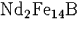-magnet. The length of its edges is 200 nm. The initial magnetization consists of two domains: The upper half of the cube is magnetized parallel to the x-axis in negative direction, the lower half in positive direction. Consequently there is a domain wall in between. It can be examined in greater detail by refining the mesh in this area.

First a new document (with the name demo) is created and initialized with the magnetization problem on the cube domain using the multigrid algorithm.

open demo cube magnetization multigrid

Then the cube is triangulated using Kuhn's method. (See figure 3 for the corners' numbering.)

element 0 1 3 7
element 0 1 5 7
element 0 2 3 7
element 0 2 6 7
element 0 4 5 7
element 0 4 6 7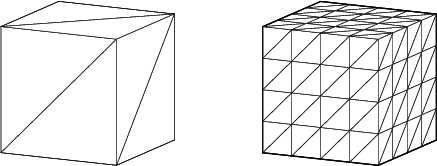After refining the whole grid two times, the cube consists of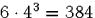tetrahedrons and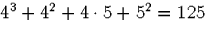vertices. The initial triangulation and the twice refined mesh are shown in figure 5.1. With impsol (cf. section 4.2.3) the two desired domains of magnetization are initialized.

The initial triangulation should be as coarse as possible, just fine enough to resolve the shape of the domain and the coefficient jumps in the problem under consideration. However, it can be expected that the grid will have to be refined at the domain wall, which will be very thin in a hard magnetic material (typically some nanometres). Therefore we start with a sufficiently fine grid. Now the files containing all information necessary for vecu can be generated:

expvertices cube.knt
expelements cube.ijk
expboundary cube.off
expsol cube.mag

Then mesh33, material2, omega33, matrix2, and vecmesh32 do the preprocessing before vecu33 can be invoked. Following vecu's calculations the results have to be imported in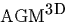.Figure 7 shows the total energy stored in the magnetization of the cube as a function of the simulation time.

impsol cube.inp
imperror cube.ind

These commands will assign to each vertex its calculated magnetization vector and to each element its estimated error.

The estimated error has to be evaluated and for each element decided if it has to be refined or not. For this task there are several estimators, which are described in section 4.2.4.

set estimator = etamax
set tolerance = 0.5 estimate

All marked elements are coloured blue (boundary faces) and green (cut plane). In figure 5 the faces of all marked elements of the left cube appear darker than the others. Then refine starts the refinement algorithm described in previous sections and creates the triangulation shown on the right of figure 5. It consists of 318 vertices and 1,312 elements. However, the new vertices contain no valid magnetization vector yet. These have to be created by interpolation with the command interpolate.

Finally, the output files can be generated as described above, the new calculation started, and the grid refined again. The result shown in figure 6 is a grid of 1,774 vertices and 8,867 elements.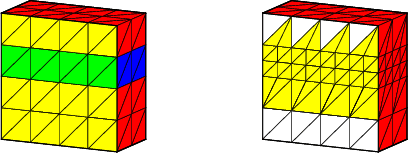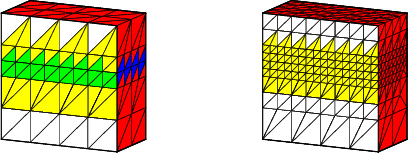Next: Simulation results Up: Application Previous: Application
Werner Scholz
12/19/1997